### Pandas 绘制线状图

 `123456789101112` ``````import pandas as pd import numpy as np import matplotlib.pyplot as plt def craw_line(): ts = pd.Series(np.random.randn(1000), index=pd.date_range("1/1/2000", periods=1000)) ts = ts.cumsum() ts.plot() plt.show() if __name__ == '__main__': craw_line() ``````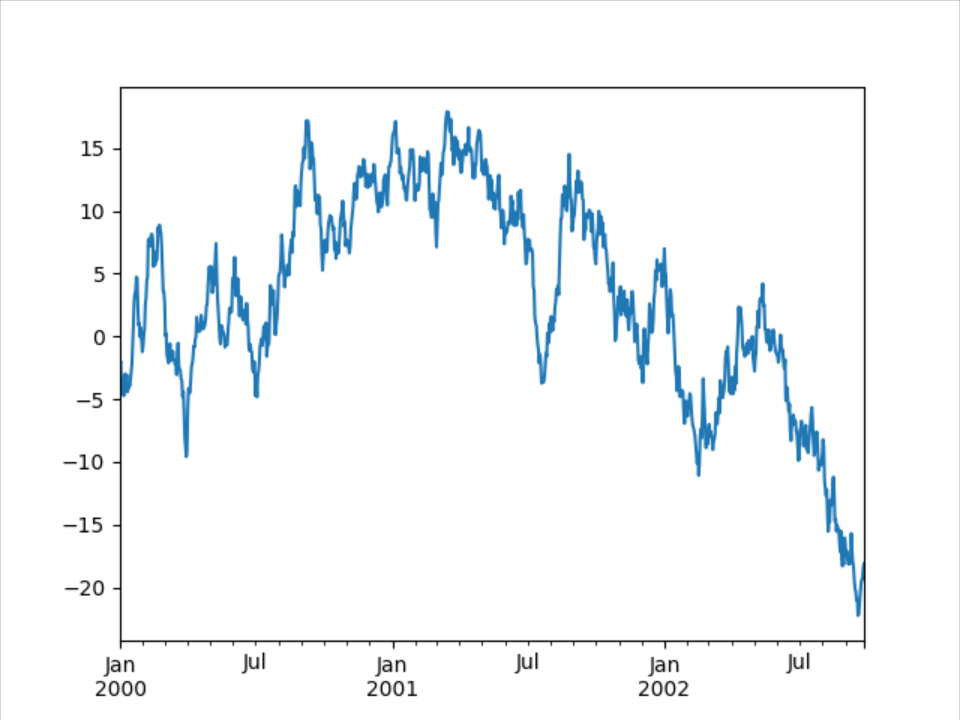`12345678910111213` ``````import pandas as pd import numpy as np import matplotlib.pyplot as plt def craw_line1(): ts = pd.Series(np.random.randn(1000), index=pd.date_range("1/1/2000", periods=1000)) df = pd.DataFrame(np.random.randn(1000, 4), index=ts.index, columns=list("ABCD")) df = df.cumsum() df.plot() plt.show() if __name__ == '__main__': craw_line1() ``````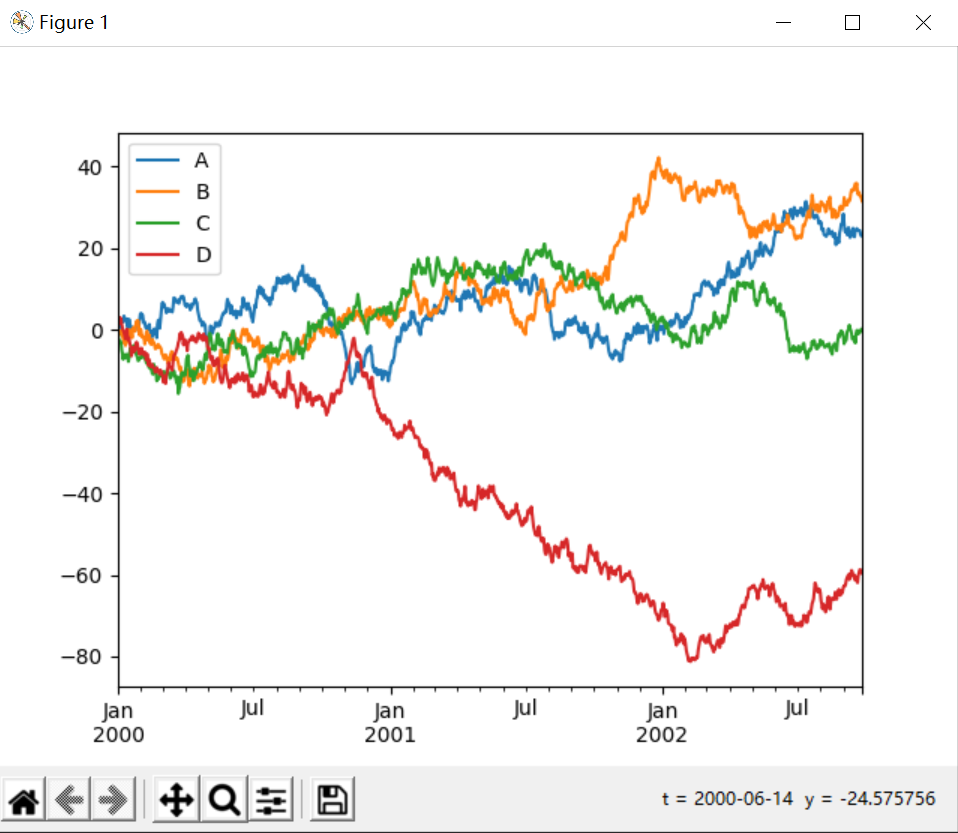### Pandas 绘制条形图

#### 绘制条形图1

 `123456789` ``````def craw_bar(): ts = pd.Series(np.random.randn(1000), index=pd.date_range("1/1/2000", periods=1000)) df = pd.DataFrame(np.random.randn(1000, 4), index=ts.index, columns=list("ABCD")) plt.figure() df.iloc.plot(kind="bar") plt.show() if __name__ == '__main__': craw_bar() ``````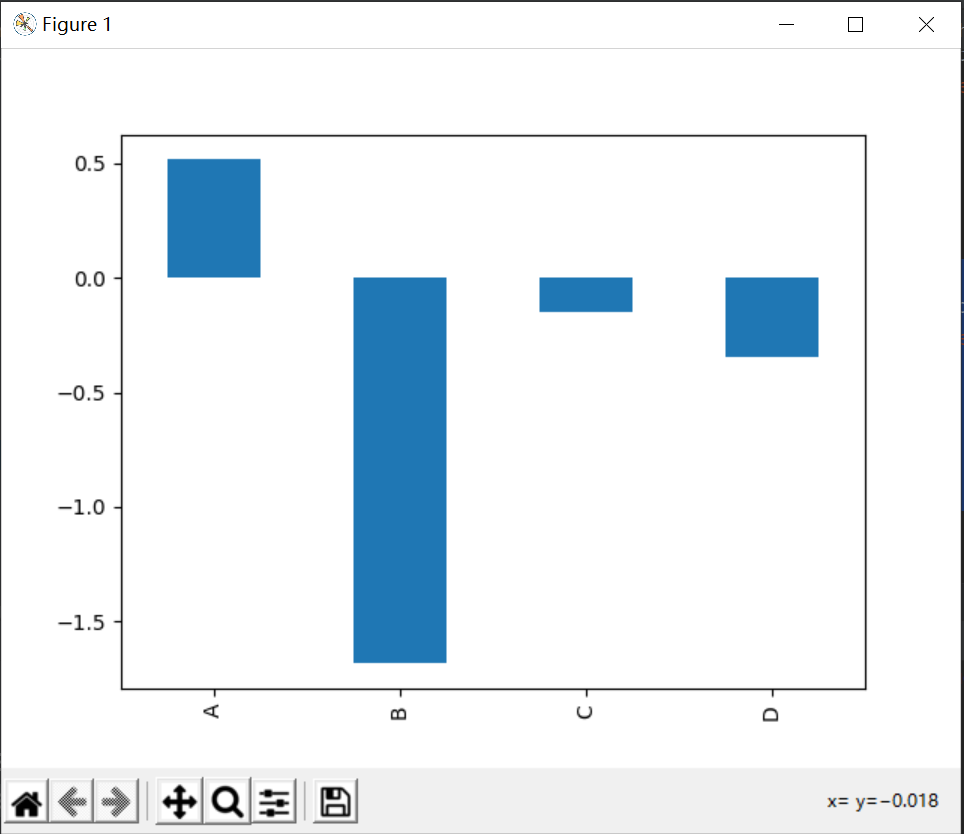#### 绘制条形图2

 `12345678` ``````def craw_bar1(): #ts = pd.Series(np.random.randn(1000), index=pd.date_range("1/1/2000", periods=1000)) df2 = pd.DataFrame(np.random.rand(10, 4), columns=["a", "b", "c", "d"]) df2.plot.bar() plt.show() if __name__ == '__main__': craw_bar1() ``````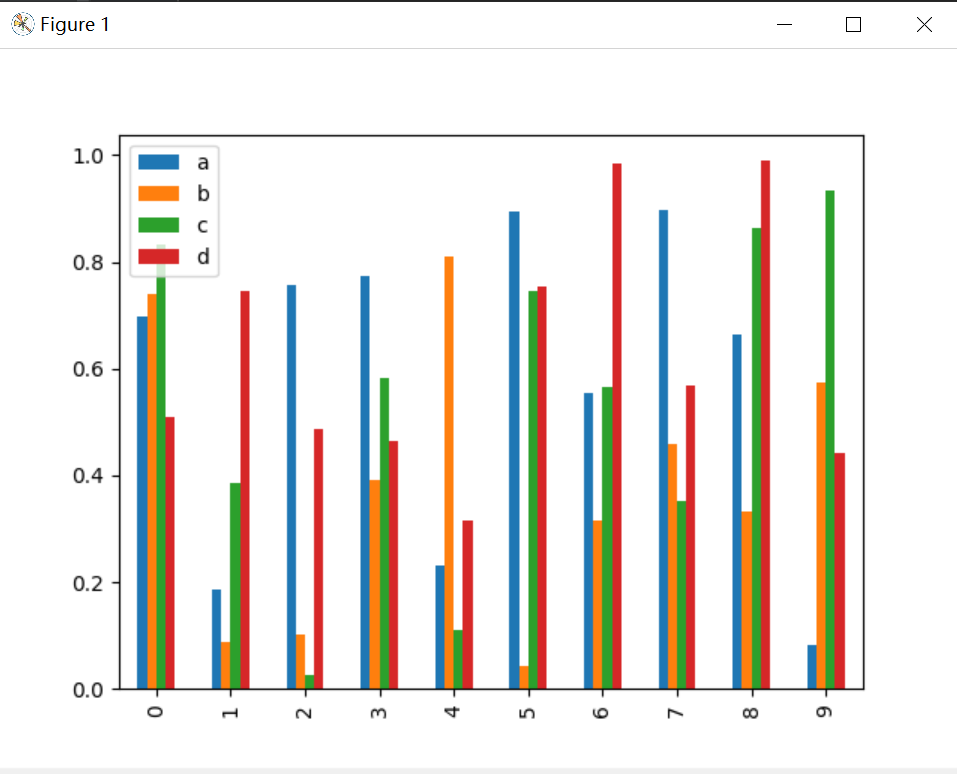`1234567` ``````def craw_bar2(): df2 = pd.DataFrame(np.random.rand(10, 4), columns=["a", "b", "c", "d"]) df2.plot.bar(stacked=True) plt.show() if __name__ == '__main__': craw_bar2() ``````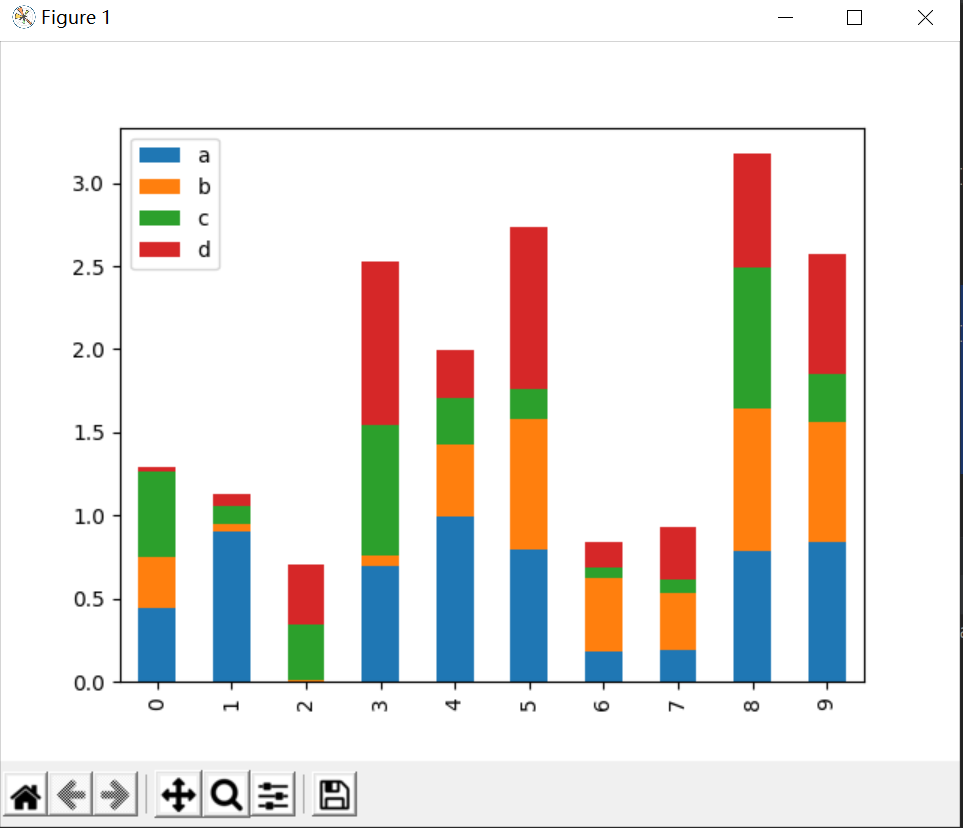`1234567` ``````def craw_bar3(): df2 = pd.DataFrame(np.random.rand(10, 4), columns=["a", "b", "c", "d"]) df2.plot.barh(stacked=True) plt.show() if __name__ == '__main__': craw_bar3() ``````### 总结